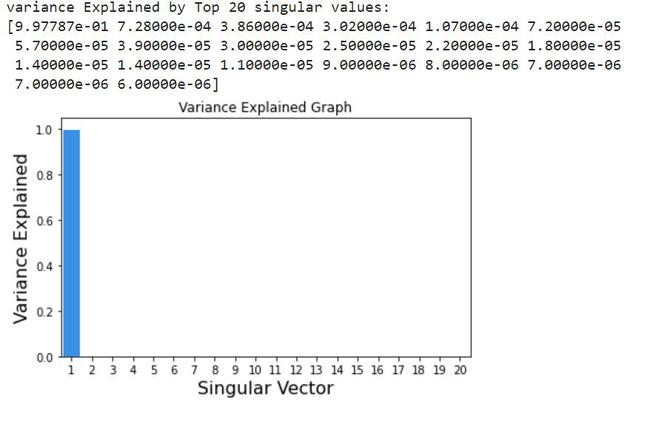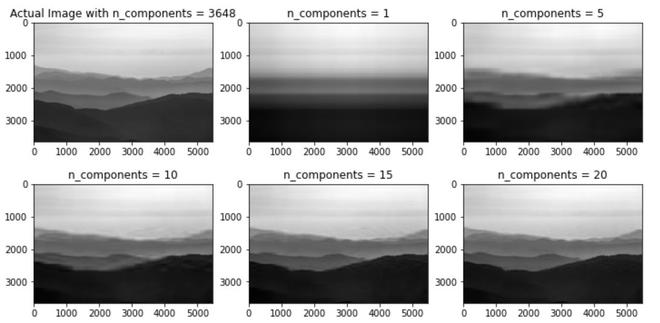Related Articles
Image Reconstruction using Singular Value Decomposition (SVD) in Python
• Last Updated : 05 Apr, 2021

Singular Value Decomposition aka SVD is one of many matrix decomposition Technique that decomposes a matrix into 3 sub-matrices namely U, S, V where U is the left eigenvector, S is a diagonal matrix of singular values and V is called the right eigenvector. We can reconstruct SVD of an image by using linalg.svd() method of NumPy module.

Syntax:

linalg.svd(matrix, full_matrices=True, compute_uv=True, hermitian=False)

Parameters:

1. matrix : A real or complex matrix of size > 2.
2. full_matrices: If True the size of u and v matrics are m x n , if False then the shape of u and v matrices are m x k , where k is non-zero values only.
3. compute_uv: Takes in boolean value to compute u and v matrices along with s matrix.
4. hermitian: By default matrix is assumed to be Hermitian if it contains real-values, this is used internally for efficiently computing the singular values.

Image Used:## Python3

 `# import module``import` `requests``import` `cv2``import` `numpy as np``import` `matplotlib.pyplot as plt`` ` `# assign and open image``url ``=` `'https://media.geeksforgeeks.org/wp-content/cdn-uploads/20210401173418/Webp-compressed.jpg'``response ``=` `requests.get(url, stream``=``True``)`` ` `with ``open``(``'image.png'``, ``'wb'``) as f:``    ``f.write(response.content)`` ` `img ``=` `cv2.imread(``'image.png'``)`` ` `# Converting the image into gray scale for faster``# computation.``gray_image ``=` `cv2.cvtColor(img, cv2.COLOR_BGR2GRAY)`` ` `# Calulating the SVD``u, s, v ``=` `np.linalg.svd(gray_image, full_matrices``=``False``)`` ` `# inspect shapes of the matrices``print``(f``'u.shape:{u.shape},s.shape:{s.shape},v.shape:{v.shape}'``)`

Output:

`u.shape:(3648, 3648),s.shape:(3648,),v.shape:(3648, 5472)`

Explanation:

The above output shape indicates that there are 3648 linearly independent eigenvectors in this image.

Now let us look at the variance of the image used over a singular vector graphically:

## Python3

 `# import module``import` `seaborn as sns`` ` `var_explained ``=` `np.``round``(s``*``*``2``/``np.``sum``(s``*``*``2``), decimals``=``6``)`` ` `# Variance explained top Singular vectors``print``(f``'variance Explained by Top 20 singular values:\n{var_explained[0:20]}'``)`` ` `sns.barplot(x``=``list``(``range``(``1``, ``21``)),``            ``y``=``var_explained[``0``:``20``], color``=``"dodgerblue"``)`` ` `plt.title(``'Variance Explained Graph'``)``plt.xlabel(``'Singular Vector'``, fontsize``=``16``)``plt.ylabel(``'Variance Explained'``, fontsize``=``16``)``plt.tight_layout()``plt.show()`

Output:Variance Explained Graph.

Explanation: The Variance Explained Graph above clearly shows that about 99.77 % of information is explained by the first eigenvector and its corresponding eigenvalues themselves. Therefore, it very much advisable to reconstruct the image with just the top few eigenvectors themselves.

In the below program based on the above discussion, we reconstruct the image using SVD:

## Python3

 `# plot images with different number of components``comps ``=` `[``3648``, ``1``, ``5``, ``10``, ``15``, ``20``]``plt.figure(figsize``=``(``12``, ``6``))`` ` `for` `i ``in` `range``(``len``(comps)):``    ``low_rank ``=` `u[:, :comps[i]] @ np.diag(s[:comps[i]]) @ v[:comps[i], :]``     ` `    ``if``(i ``=``=` `0``):``        ``plt.subplot(``2``, ``3``, i``+``1``),``        ``plt.imshow(low_rank, cmap``=``'gray'``),``        ``plt.title(f``'Actual Image with n_components = {comps[i]}'``)``     ` `    ``else``:``        ``plt.subplot(``2``, ``3``, i``+``1``),``        ``plt.imshow(low_rank, cmap``=``'gray'``),``        ``plt.title(f``'n_components = {comps[i]}'``)`

Output:Image Reconstructed using SVD.

Explanation:

1. Though the 1st eigenvector contains 99.77% of information reconstructing an image solely from it does not give a clear picture.
2. Using the top 15 vectors for the image reconstruction gives a good enough approximation. Also out of 3648 vectors which is a massive decrease in computation and also it compresses the image.

Attention geek! Strengthen your foundations with the Python Programming Foundation Course and learn the basics.

To begin with, your interview preparations Enhance your Data Structures concepts with the Python DS Course. And to begin with your Machine Learning Journey, join the Machine Learning – Basic Level Course

My Personal Notes arrow_drop_up